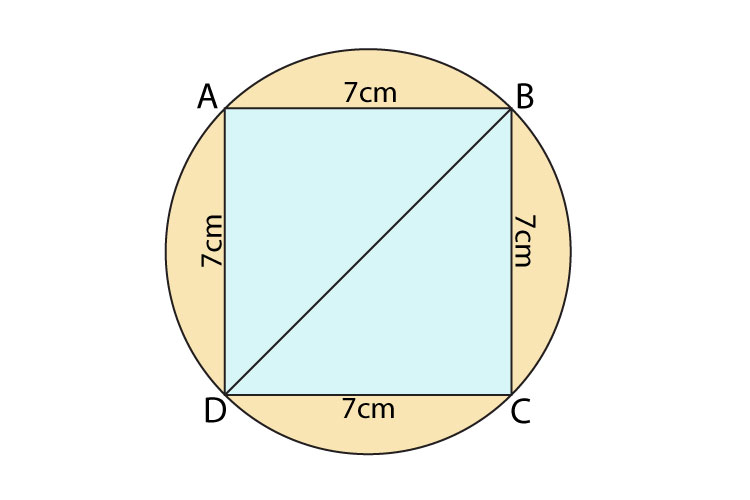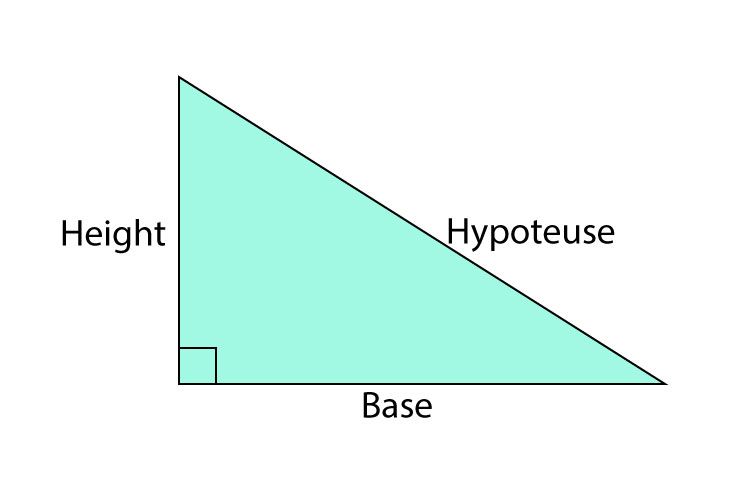# A,B,C and D are points on a circle. ABCD is a square of side 7 cm. Work out the total area of the shaded regions. Give your answer correct to the nearest whole number.BD= diameter of the circle

As shown in the diagram and the question, the square is inscribed inside the circle and we are supposed to find the area of the shaded blue region that doesn’t include the area of the square.

Step 1: Find the area of the square

Area= Length × width

= 7×7

= 49 cm²

Step 2: Find the diameter of the circle

In order to calculate the area of a circle, we need the measurement of the radius. However, before calculating the radius, we will need to find the diameter, which will aid us in finding the value of the radius and the area of the circle.

Since, we know that the shape inside the circle is a square, which has equal sides of 7cm, so we will be using the Pythagoras Theorem to find the length of the diagonal. The length of the diagonal will be equal to the diameter because the diameter is represented by the distance between two points on the circumference that passes through the centre of the circle, which is given by the length of BD as shown in the diagram above.

Pythagoras Theorem:In our case, height = BC = 7 cm and base = DC = 7 cm.  Now substitute these values in the pythagoras theorem formula to find the value of the hypotenuse, which is BD.

BC² + CD² = BD²

(7)² + (7)² = BD²

49 + 49 = BD²

√98 = BD

9.899 cm = BD ⇒ Diameter of the circle

In order to calculate the radius, we will be using the diameter. The formula for the diameter is:

= 9.899 ÷ 2

= 4.949 cm

Step 4: Find the area of the circle

Area of the circle= πr²

= π × (4.949)²

= 76.67 cm²

Step 5: Find the area of the shaded region

Area of the shaded region = Area of the circle - Area of the square

= 76.67 - 49

= 27.66 cm² ≃ 28 cm²